### 大众(进口) 夏朗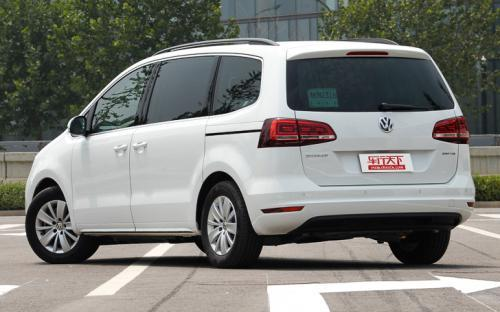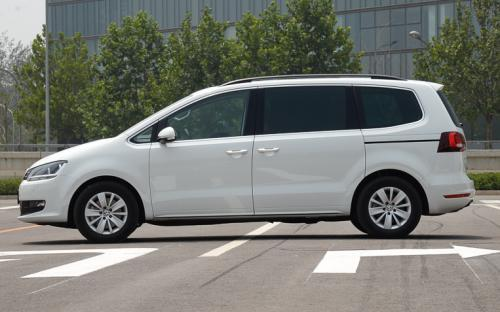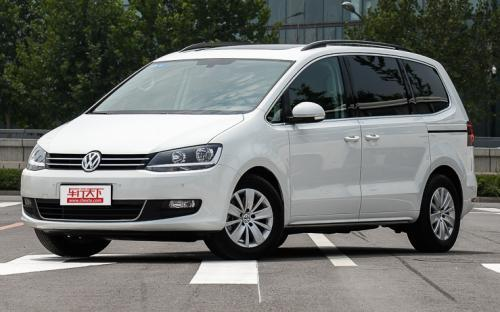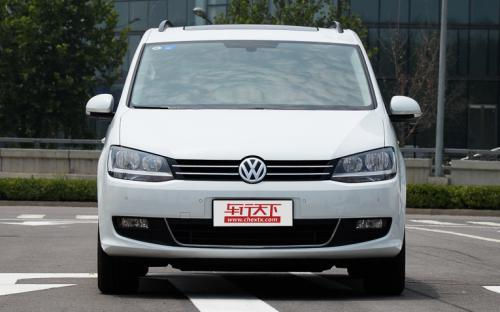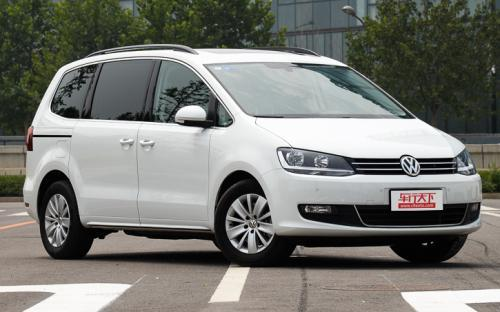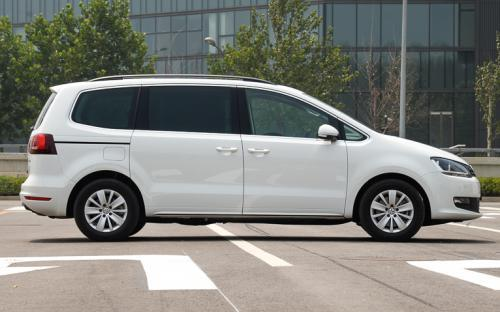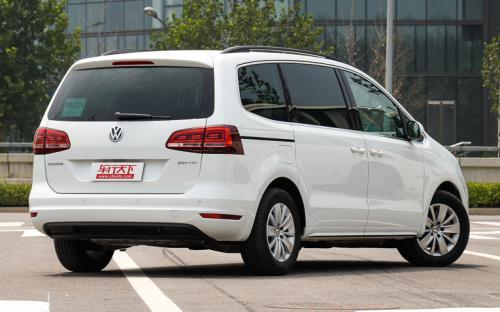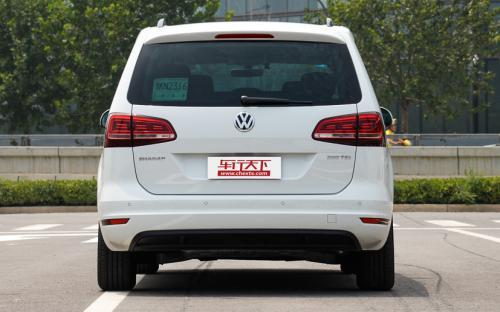16 种颜色可选2016款最低售价：26.98 万元起

4854(mm)1904(mm)1740(mm)##### 配置亮点：
• 胎压监测装置

• ISOFIX儿童座椅接口

• 车身稳定控制(ESC/ESP/DSC等)

• 电动天窗

• 定速巡航

• 后倒车雷达

• 真皮座椅

• GPS导航系统

• 氙气大灯

• 后视镜加热

• 提交
2016款 280TSI 乐享型 (167张)
• 2016款 280TSI 乐享型 (167张)
• 2016款 380TSI 暖心红限量版 (118张)
• 大众(进口) 夏朗 绕车实拍• 大众(进口) 夏朗 在售车型

排量 车型 厂商指导价 本地最低报价 购车工具
1.4T
280TSI 乐享型 6挡双离合
26.98万
26.98万

29.58万
29.58万

380TSI 尊享型 6挡双离合
34.68万
34.68万

30.88万
30.88万
2.0T
280TSI 乐享型 6挡双离合
26.98万
26.98万

29.58万
29.58万

380TSI 尊享型 6挡双离合
34.68万
34.68万

30.88万
30.88万

大众(进口) 夏朗 经销商

查看更多 >>

### 大众(进口) 夏朗 动力加速

夏朗 0-100公里加速时间分布在 0.0-秒 属于 超跑级

动力级别 加速时间 车型

大众(进口) 夏朗 视频

大众(进口) 夏朗 新闻资讯

# 大众新款夏朗上市 售价26.98-34.68万

上市新车 超过8313次关注

在今年开幕的深港澳车展上，进口大众的新款夏朗正式上市，新车在4月底推出了限量版车型，动力系统方面变化较大，取消了原来的1.8TSI发动机，提供1.4TSI和2.0TSI发动...

# 上海大众有90家经销商开售大众进口车

技术 超过5581次关注

上海大众汽车大众进口车型尊荣品鉴会活动在苏州河畔的上海游艇会正式举办，该活动的主旨是向大家再次宣布上海大众汽车VW品牌4S店将开始正式销售大众进口汽车，先期...

# 夏朗对比体验Alhambra 定位完全不同

评测 超过8616次关注

对很多网友来说，大众夏朗和西雅特Alhambra应该算是两个比较陌生的名字，尤其是后者，老实说在此次车展前甚至是我也对它没什么概念。在查阅了一些资料后我得知西雅...

# 进口大众多款车搭载车联网系统 4年免费

新闻 超过5115次关注

大众进口汽车正式推出了车联网服务。该服务将搭载于进口Tiguan、甲壳虫、尚酷、高尔夫R敞篷轿车、夏朗、迈腾旅行轿车、迈腾四驱旅行车等多款大众进口车型上，并为用...

# 大众夏朗2.0T车型增配 售32.58万元起

新闻 超过5217次关注

近日，我们从北京经销商处获得消息称，大众夏朗2.0T车型将会在配置上有所调整，同时新车的价格也将发生变化，新车的售价为32.58万元-42.30万元。

# 大众CUP版多款新车型发布 细节设计有变

新闻 超过5179次关注

近日，大众在海外市场发布了一系列车型的CUP版本，涉及车型包括UP！、高尔夫、高尔夫Variant、高尔夫敞篷版、甲壳虫硬顶和敞篷版、EOS、途安、夏朗以及Tiguan车型，...

猜你喜欢

﻿
• 快速找车
• 选择品牌
• 选择品牌
• A  奥迪
• A  阿斯顿·马丁
• A  阿尔法·罗密欧
• B  宝沃
• B  布加迪
• B  巴博斯
• B  保时捷
• B  宾利
• B  奔驰
• B  宝马
• B  本田
• B  别克
• B  标致
• B  比亚迪
• B  宝骏
• B  北汽制造
• B  北汽新能源
• B  北汽幻速
• B  北汽威旺
• B  北京汽车
• B  奔腾
• B  北汽绅宝
• C  长安
• C  长安商用
• C  长城
• C  昌河
• D  大众
• D  道奇
• D  DS
• D  东南
• D  东风风神
• D  东风风行
• D  东风小康
• D  东风风度
• D  东风
• F  福特
• F  丰田
• F  菲亚特
• F  法拉利
• F  福田
• F  福迪
• F  福汽启腾
• G  观致
• G  广汽传祺
• G  广汽吉奥
• G  GMC
• H  红旗
• H  汉腾汽车
• H  哈弗
• H  哈飞
• H  海格
• H  海马
• H  华颂
• H  黄海
• H  华泰
• H  恒天
• J  吉利汽车
• J  捷豹
• J  Jeep
• J  江淮
• J  江铃
• J  金杯
• J  九龙
• J  金旅
• K  凯翼
• K  凯迪拉克
• K  克莱斯勒
• K  科尼塞克
• K  卡威
• K  开瑞
• L  路虎
• L  林肯
• L  劳斯莱斯
• L  兰博基尼
• L  雷克萨斯
• L  铃木
• L  雷诺
• L  理念
• L  力帆
• L  莲花汽车
• L  猎豹
• L  路特斯
• L  陆风
• M  马自达
• M  MG
• M  MINI
• M  玛莎拉蒂
• M  摩根
• M  迈凯轮
• N  纳智捷
• O  欧宝
• O  讴歌
• O  欧朗
• Q  奇瑞
• Q  起亚
• Q  启辰
• R  日产
• R  荣威
• R  瑞麒
• S  三菱
• S  斯威汽车
• S  萨博
• S  smart
• S  斯柯达
• S  斯巴鲁
• S  思铭
• S  双龙
• S  上汽大通
• S  双环
• T  特斯拉
• T  腾势
• W  沃尔沃
• W  五菱汽车
• W  五十铃
• W  威兹曼
• W  威麟
• X  现代
• X  雪佛兰
• X  雪铁龙
• X  西雅特
• Y  一汽
• Y  英菲尼迪
• Y  英致
• Y  依维柯
• Y  野马汽车
• Y  永源
• Z  众泰
• Z  中华
• Z  中兴
• Z  知豆
• 选择车系
• 选择车系
• 车型对比
• 选择品牌
• 选择品牌
• A  奥迪
• A  阿斯顿·马丁
• A  阿尔法·罗密欧
• B  宝沃
• B  布加迪
• B  巴博斯
• B  保时捷
• B  宾利
• B  奔驰
• B  宝马
• B  本田
• B  别克
• B  标致
• B  比亚迪
• B  宝骏
• B  北汽制造
• B  北汽新能源
• B  北汽幻速
• B  北汽威旺
• B  北京汽车
• B  奔腾
• B  北汽绅宝
• C  长安
• C  长安商用
• C  长城
• C  昌河
• D  大众
• D  道奇
• D  DS
• D  东南
• D  东风风神
• D  东风风行
• D  东风小康
• D  东风风度
• D  东风
• F  福特
• F  丰田
• F  菲亚特
• F  法拉利
• F  福田
• F  福迪
• F  福汽启腾
• G  观致
• G  广汽传祺
• G  广汽吉奥
• G  GMC
• H  红旗
• H  汉腾汽车
• H  哈弗
• H  哈飞
• H  海格
• H  海马
• H  华颂
• H  黄海
• H  华泰
• H  恒天
• J  吉利汽车
• J  捷豹
• J  Jeep
• J  江淮
• J  江铃
• J  金杯
• J  九龙
• J  金旅
• K  凯翼
• K  凯迪拉克
• K  克莱斯勒
• K  科尼塞克
• K  卡威
• K  开瑞
• L  路虎
• L  林肯
• L  劳斯莱斯
• L  兰博基尼
• L  雷克萨斯
• L  铃木
• L  雷诺
• L  理念
• L  力帆
• L  莲花汽车
• L  猎豹
• L  路特斯
• L  陆风
• M  马自达
• M  MG
• M  MINI
• M  玛莎拉蒂
• M  摩根
• M  迈凯轮
• N  纳智捷
• O  欧宝
• O  讴歌
• O  欧朗
• Q  奇瑞
• Q  起亚
• Q  启辰
• R  日产
• R  荣威
• R  瑞麒
• S  三菱
• S  斯威汽车
• S  萨博
• S  smart
• S  斯柯达
• S  斯巴鲁
• S  思铭
• S  双龙
• S  上汽大通
• S  双环
• T  特斯拉
• T  腾势
• W  沃尔沃
• W  五菱汽车
• W  五十铃
• W  威兹曼
• W  威麟
• X  现代
• X  雪佛兰
• X  雪铁龙
• X  西雅特
• Y  一汽
• Y  英菲尼迪
• Y  英致
• Y  依维柯
• Y  野马汽车
• Y  永源
• Z  众泰
• Z  中华
• Z  中兴
• Z  知豆
• 选择车系
• 选择车系
• 选择车型
• 选择车型
• 意见反馈